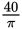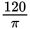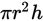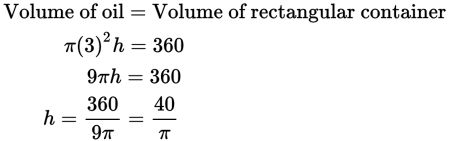# SAT Math Multiple Choice Question 491: Answer and Explanation

### Test Information

Question: 491

11. Crude oil is being transferred from a full rectangular storage container with dimensions 4 meters by 9 meters by 10 meters into a cylindrical transportation container that has a diameter of 6 meters. What is the minimum possible length for a transportation container that will hold all of the oil?

• A. 40π
• B.• C. 60π
• D.Explanation:

B

Difficulty: Medium

Category: Additional Topics in Math / Geometry

Strategic Advice: Think about this question logically before you start writing things down—after it's transferred, the volume of the oil in the cylindrical container will be the same volume as the rectangular container, so you need to set the two volumes equal and solve for h.

Getting to the Answer: The volume of the rectangular container is 4 × 9 × 10, or 360 cubic meters. The volume of a cylinder equals the area of its base times its height, or. Because the diameter is 6 meters, the radius, r, is half that, or 3 meters. Now we're ready to set up an equation and solve for h (which is the height of the cylinder or, in this case, the length of the transportation container):